The K5 Learning Blog urges parents to be pro-active in helping their children reach their full academic potential.

K5 Learning
provides free worksheets, workbooks and an online reading and math program for kindergarten to grade 5 students.# Step by Step Guide to Multiplying 3-Digit by 2-Digit Numbers

When students learn to multiply large numbers, such as 3-digit by 2-digit numbers, they learn to multiply them in columns. They usually start learning this method in grade 4 and repeat it in grade 5 and 6.

## Multiplying 3-digit by 2-digit numbers in columns

Let’s say you want to multiply 372 by 43.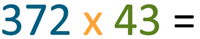The first thing you do is place the large number (the 3-digit number) above the smaller number (the 2-digit number).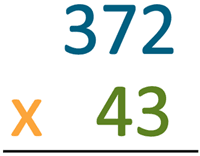Make sure you line them up so the ones places are in a column, and the tens places are in a column.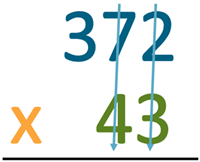Now that we have the numbers lined up, it’s time to start multiplying.

We start with multiplying the ones place column of the lower number: 3 into 372.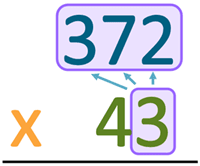Now we start with the ones places in each of the 2- and 3-digit numbers. In our example that is 3 x 2. 3 x 2 = 6.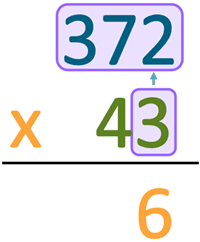Next, we multiply the 3 to the tens number in the top number. In our example, it’s 3 x 7. 3 x 7 = 21. Now this a two-digit number, so we need to pay special attention to where the numbers go in the columns. The ten number in our sum: the 2 will need to be carried. Some people put that number on the side, but a better place for the carried number is on top of the hundreds column. That way, you won’t forget it.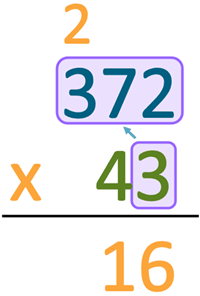In our next equation, we won’t forget that the 2 needs to be added to the sum.

Now, we multiply the hundreds number in the top number by the ones number below. In our example, that is: 3 x 3. 3 x 3 = 9. We also need to add the carried 2. 9 + 2 = 11. As this is the last number on this line, we put the 11 under the line.

We also usually put a line through the carried number once used, so as not to confuse ourselves when we work out the tens number multiplications.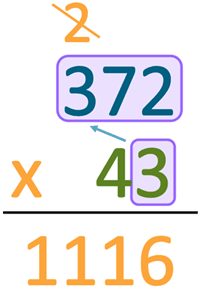Phew, we’re halfway there.

Now, we do the same for the tens. First, let’s add a zero down the ones column before we move onto the tens column. This will help us not get confused over where the numbers for the tens multiplications start on this new line.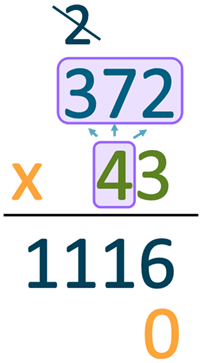Multiply the bottom tens place number with the ones place number in the top number. In our example, that’s 4 x 2. 4 x 2 = 8.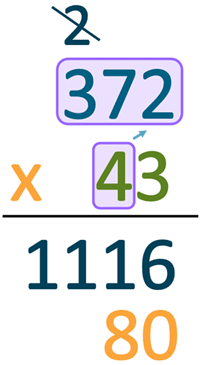Next is the tens places turn. We multiply 4 x 7 in our example. 4 x 7 = 28. Again, we have a number that needs to be carried – the 2.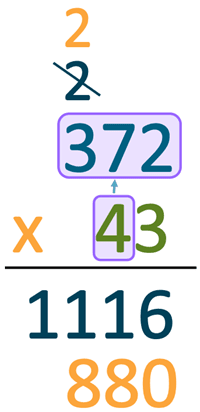Finally, we need to multiple the tens value number on the bottom number with the hundreds place number in the top number. That is 4 x 3. 4 x 3 = 12. Let’s not forget the carried number: 12 + 2 = 14.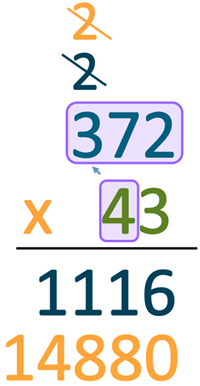From here on, we add the two lines of sums: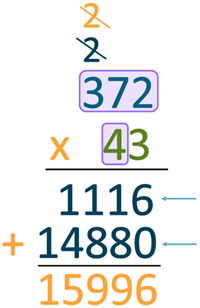The sum of 372 x 43 is 15,996.

## Worksheets for practicing 2-digit by 3-digit numbers practice

We have a number of worksheets for students to practice multiplication in columns.